# How to Calculate and Solve for Depth of Available Water | Irrigation Water Requirement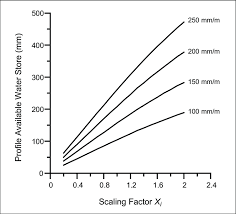The image above represents depth of available water.

To compute for depth of available water, three essential parameters are needed and these parameters are Apparent Specific Gravity of the Soil (s), Depth of the Root Zone of the Plant (d) and Water Content (m).

The formula for calculating depth of available water:

dw = s . d . m

Where:

dw = Depth of Available Water
s = Apparent Specific Gravity of the Soil
d = Depth of the Root Zone of the Plant
m = Water Content

Let’s solve an example;
Find the depth of available water when the apparent sepcific gravity of the soil is 10, the depth of the root zone of the plant is 8 and the water content is 4.

This implies that;

s = Apparent Specific Gravity of the Soil = 10
d = Depth of the Root Zone of the Plant = 8
m = Water Content = 4

dw = s . d . m
dw = (10) . (8) . (4)
dw = 320

Therefore, the depth of available water is 320.

Calculating for the Apparent Specific Gravity of the Soil when the Depth of Available Water, the Depth of the Root Zone of the Plant and the Water content is Given.

s =dw / d . m

Where;

s = Apparent Specific Gravity of the Soil
dw = Depth of Available water
d = Depth of the Root Zone of the Plant
m = Water Content

Let’s Solve an example;
Find the apparent specific gravity of the soil when the depth of available water is 60, the depth of the root zone of the plant is 10 and the water content is 3.

This implies that;

dw = Depth of Available water = 60
d = Depth of the Root Zone of the Plant = 10
m = Water Content = 3

s =dw / d . m
s =60 / 10 . 3
s =60 / 30
s = 2

Therefore, the apparent specific gravity of the soil is 2.

Calculating for the Depth of the Root Zone of the Plant when the Depth of Available Water, the Apparent Specific Gravity of the Soil and the Water content is Given.

d = dw / s . m

Where;

d = Depth of the Root Zone of the Plant
dw = Depth of Available Water
s = Apparent Specific Gravity of the Soil
m = Water Content

Let’s Solve an example;
Find the depth of the root zone of the plant when the depth of available water is 30, the apparent specific gravity of the soil is 2 and the water content is 5.

This implies that;

dw = Depth of Available Water = 30
s = Apparent Specific Gravity of the Soil = 2
m = Water Content = 5

d = dw / s . m
d = 30 / 2 . 5
d = 30 / 10
d = 3

Therefore, the depth of the root zone of the plant is 3.

Calculating for the Water content when the Depth of Available Water, the Depth of the Root Zone of the Plant and the Apparent Specific Gravity of the Soil is Given.

m = dw / s . d

Where;

m = Water Content
dw = Depth of Available Water
s = Apparent Specific Gravity of the Soil
d = Depth of the Root Zone of the Plant

Let’s Solve an example;
Find the water content when the depth of available water is 36, the apparent specific gravity of the soil is 4 and the depth of the root zone of the plant is 3.

This implies that;

dw = Depth of Available Water = 36
s = Apparent Specific Gravity of the Soil = 4
d = Depth of the Root Zone of the Plant = 3

m = dw / s . d
m = 36 / 4 . 3
m = 36 / 12
m = 3

Therefore, the water content is 3.

Nickzom Calculator – The Calculator Encyclopedia is capable of calculating the depth of available water.

To get the answer and workings of the depth of available water using the Nickzom Calculator – The Calculator Encyclopedia. First, you need to obtain the app.

You can get this app via any of these means:

To get access to the professional version via web, you need to register and subscribe for NGN 2,000 per annum to have utter access to all functionalities.
You can also try the demo version via https://www.nickzom.org/calculator

Apple (Paid) – https://itunes.apple.com/us/app/nickzom-calculator/id1331162702?mt=8
Once, you have obtained the calculator encyclopedia app, proceed to the Calculator Map, then click on Agricultural under Engineering.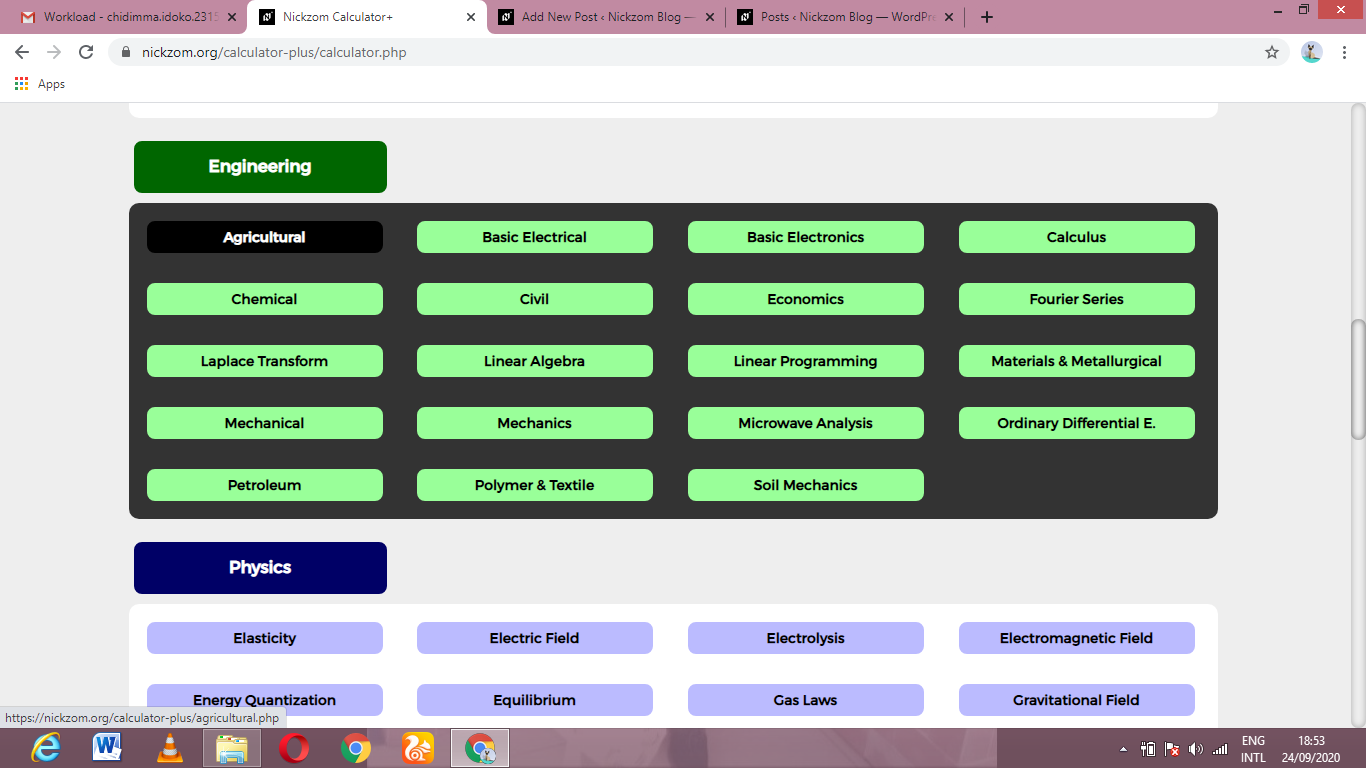Now, Click on Irrigation Water Requirement under Agricultural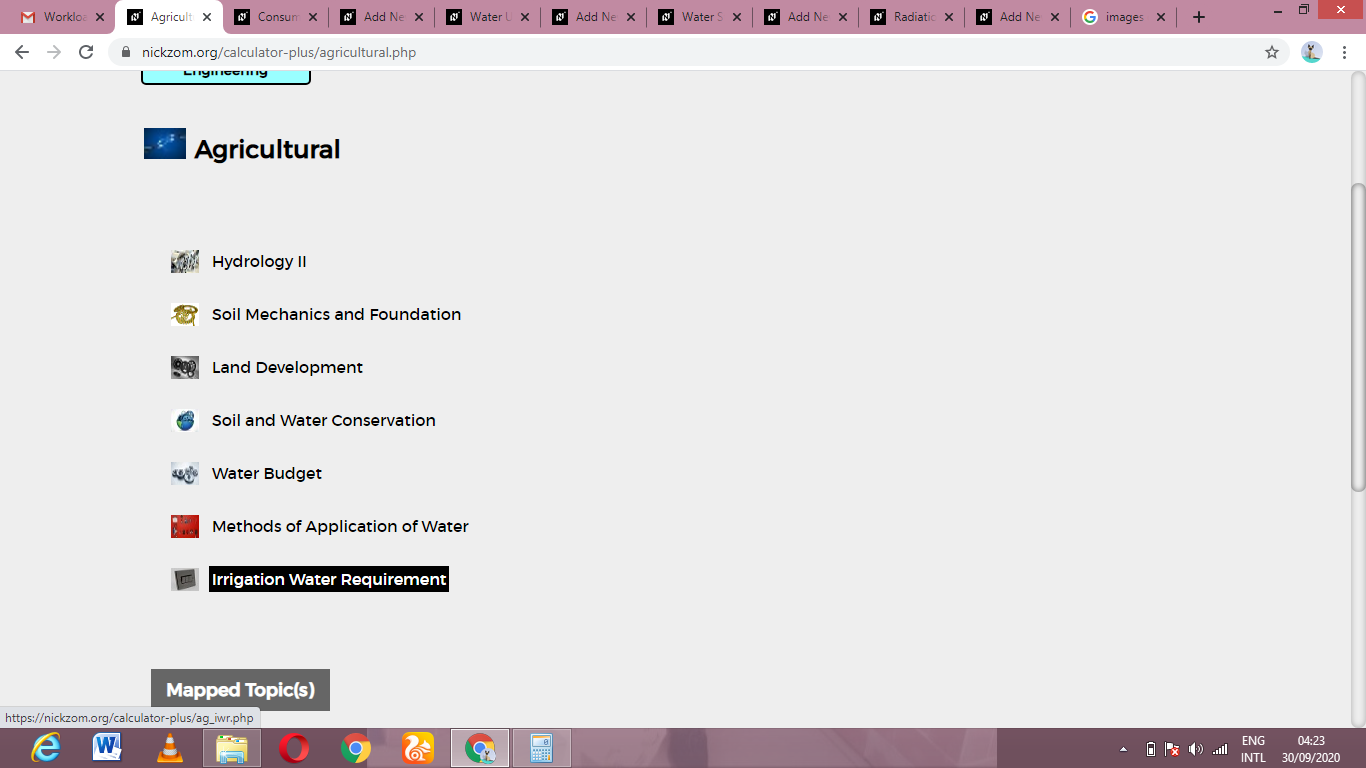Now, Click on Depth of Available Water under Irrigation Water Requirement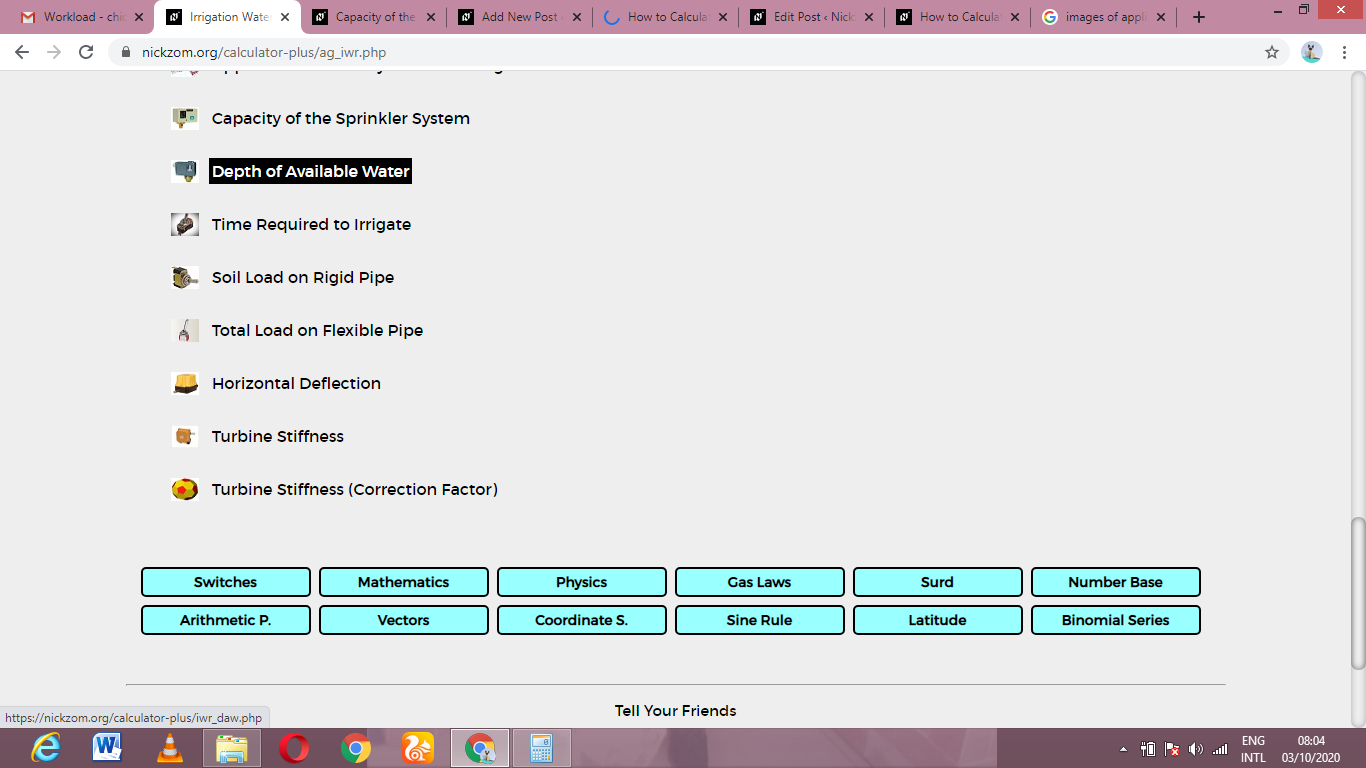The screenshot below displays the page or activity to enter your values, to get the answer for the depth of available water according to the respective parameters which is the Apparent Specific Gravity of the Soil (s), Depth of the Root Zone of the Plant (d) and Water Content (m).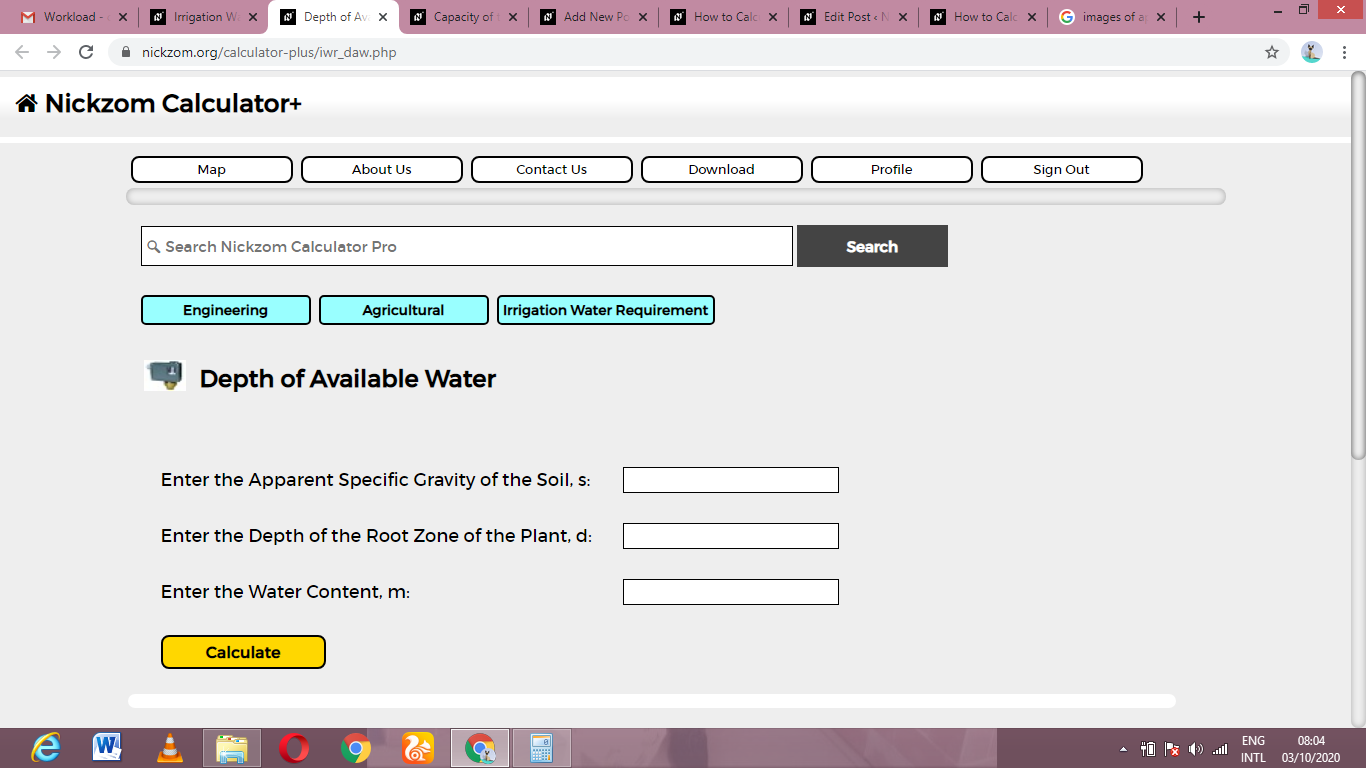Now, enter the values appropriately and accordingly for the parameters as required by the Apparent Specific Gravity of the Soil (s) is 10, Depth of the Root Zone of the Plant (d) is 8 and Water Content (m) is 4.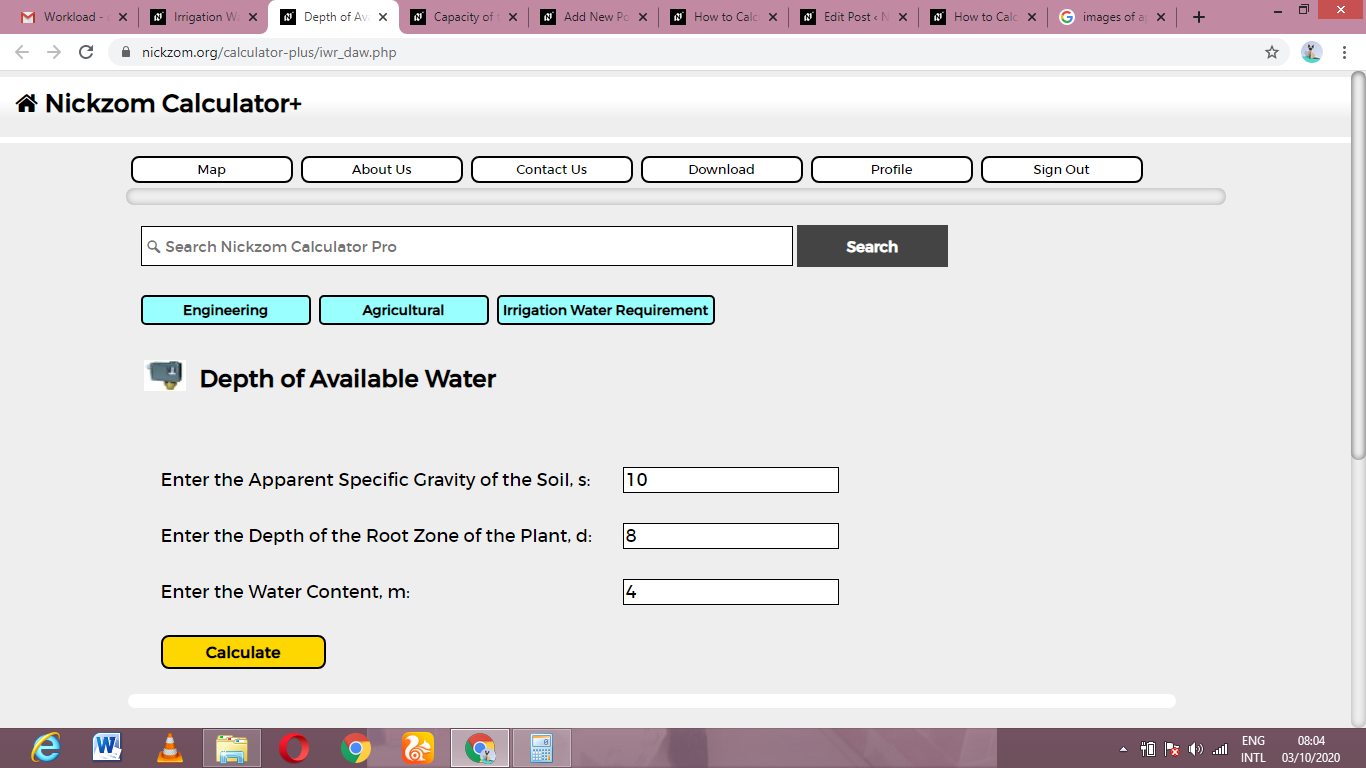Finally, Click on Calculate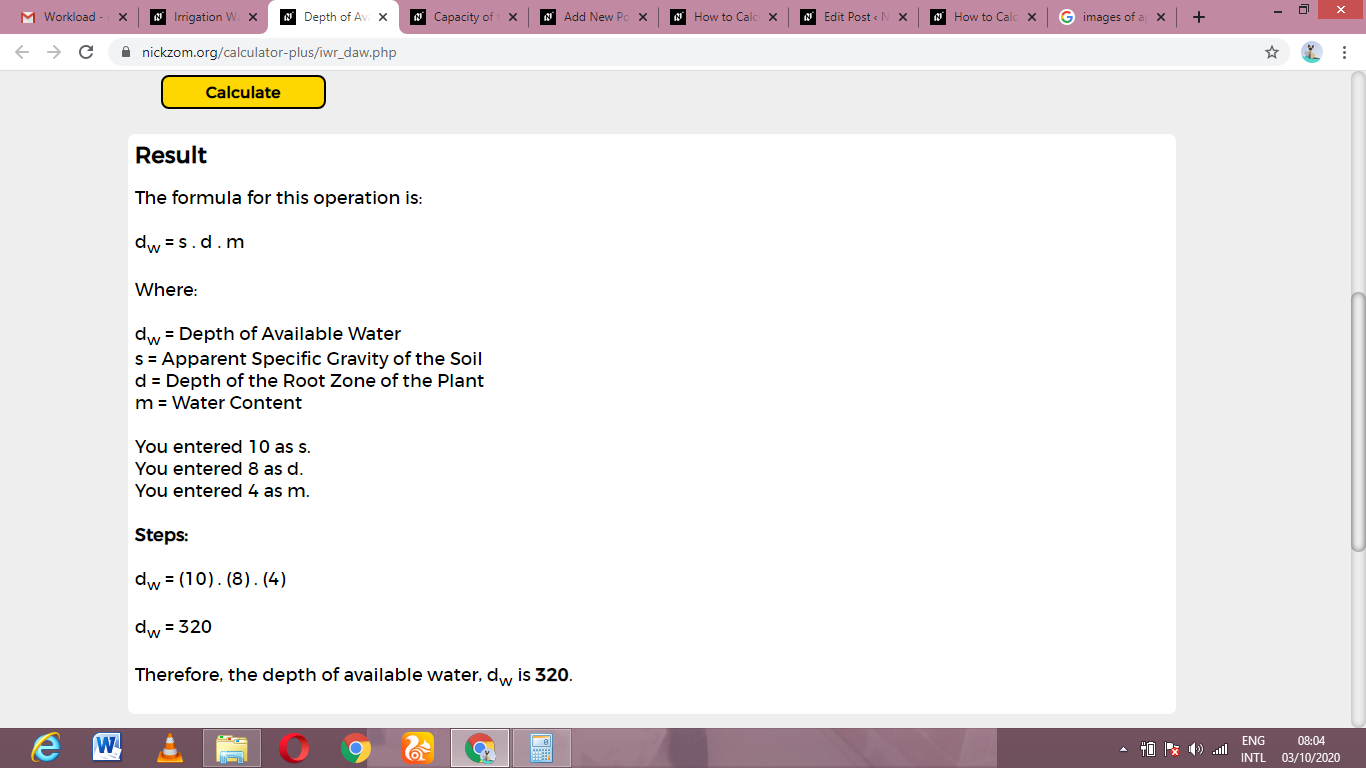As you can see from the screenshot above, Nickzom Calculator– The Calculator Encyclopedia solves for the depth of available water and presents the formula, workings and steps too.